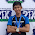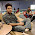## Objective Questions from Power Electronics Circuits

 Thermal runaway is not possible in FET because as the temperature of FET increases
A. The mobility decreases
B. The transconductance increases
C. The drain current increases
D. None of the above

 The ripple frequency from a full wave rectifier is
A.  Twice that from a half wave circuit
B.  The same as that from a half wave circuit
C.  Half that from a half wave circuit
D.  1/4 that from a half wave circuit

 In a full-wave rectifier using two ideal diodes,Vdc & Vm are the dc & peak values of the voltage respectively across a resistive load. If PIV is the peak inverse voltage of the diode,then the appropriate relationships for the rectifier is
A. Vdc=Vm/π,PIV=2Vm
B. Vdc=2Vm/π,PIV=2Vm
C. Vdc=2Vm/π,PIV=Vm
D. Vdc=Vm/π,PIV=Vm

 The output of a rectifier circuit without filter is
A.  50 Hz AC
B.  Smooth DC
C.  Pulsating DC
D.  60 hz AC

 An advantage of full wave bridge rectification is
A.  It uses the whole transformer secondary for the entire ac input cycle
B.  It costs less than other rectifier types
C.  It cuts off half of the ac wave cycle
D.  It never needs a filter

 The best rectifier circuit for the power supply designed to provide high power at low voltage is
A.  Half wave arrangement
B.  Full wave, center tap arrangement
C.  Quarter wave arrangement
D.  Voltage doubler arrangement

 If a half wave rectifier is used with 165Vpk AC input, the effective dc output voltage is
A.  Considerably less than 165V
B.  Slightly less than 165V
C.  Exactly 165V
D.  Slightly more than 165V

 If a full wave bridge circuit is used with a transformer whose secondary provides 50Vrms, the peak voltage that occurs across the diodes in the reverse direction is approximately
A.  50 Vpk
B.  70 Vpk
C.  100 Vpk
D.  140 Vpk

 The main disadvantage of voltage doubler power supply circuit is
A.  Excessive current
B.  Excessive voltage
C.  Insufficient rectification
D.  Poor regulation under heavy loads

 A source follower using an FET usually has a voltage gain which is
A. Greater than +100
B. Slightly less than unity but positive
C. Exactly unity but negative
D. About -10
 A metal oxide varistor (MOV) is used for protecting
(a) Gate circuit against overcurrents
(b) Gate circuit against overvoltages
(c) Anode circuit against overcurrents
(d) Anode circuit against overvoltages
OPTIONS:
1) A
2) A, B
3) C, D
4) D
5) None of the above options

 In a dual converter, the circulating current [Gate 1997]

(a) Allows smooth reversal of load current, but increases the response time
(b) Does not allow smooth reversal of load current, but reduces the response time
(c) Allows smooth reversal of load current with improved speed of load response
(d) Follows only if there is no interconnecting inductor

 The snubber circuit connected across an SCR is to

(a) Suppress dv/dt
(b) Increase dv/dt
(c) Decrease dv/dt
(d) Keep transient overvoltage at a constant value

 The object of connecting resistance and capacitance across gate circuit is to protect the SCR gate against

(a) Overvoltages
(b) dv/dt
(c) Noise signals
(d) Over currents

Thanks for reading....
8:08 PM

#### 2 comments:

1.I think the answer to question 10 is incorrect. Source-follower in FET is has similar gain characteristics to emitter-follower in BJT, which is less than unity but positive.

1.